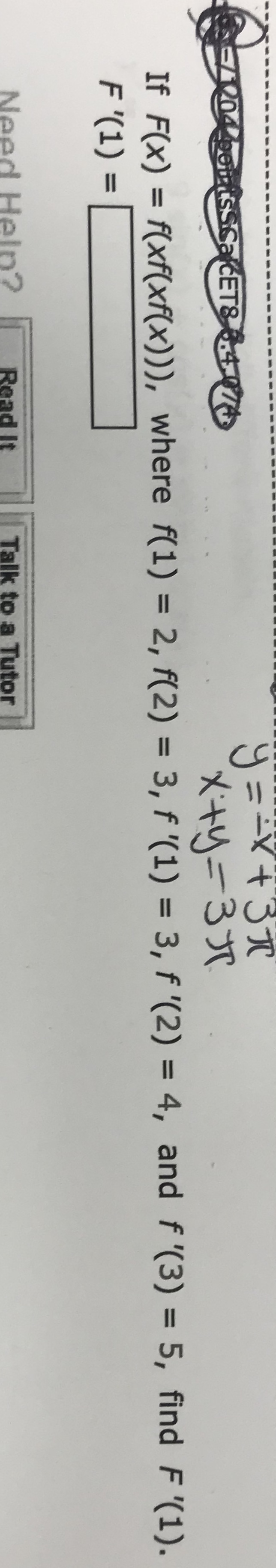# ETIf Fx) xf(xf(x))), where f(1) - 2, f(2) 3, f '(1) 3, f '(2) 4, and f (3) 5, find F (1).Need Heln?Read Itto a

Question
16 viewshelp_outlineImage TranscriptioncloseET If Fx) xf(xf(x))), where f(1) - 2, f(2) 3, f '(1) 3, f '(2) 4, and f (3) 5, find F (1). Need Heln? Read It to a fullscreen
check_circle

Step 1

The given function is F(x) = f ( x f (x f (x))).

Differentiate the function F(x) = f ( x f (x f (x)) with respect to x.

Let us assume u = x f (x f (x) ) then F(x) = f (u).

Step 2

Obtain the value of u (x) = x f (x f (x) ).

Step 3

Use the initial conditions to find the...

### Want to see the full answer?

See Solution

#### Want to see this answer and more?

Solutions are written by subject experts who are available 24/7. Questions are typically answered within 1 hour.*

See Solution
*Response times may vary by subject and question.
Tagged in

### Functions